11  Sums and quotients

Paré’s theorem, that any elementary topos has the monadic property [Par74], which originally inspired abstract Stone duality, was itself motivated by the simple way that it affords for constructing colimits. So we conclude this paper with applications of the comprehension calculus to coproducts and coequalisers.

Example 11.1 The idea of Stone duality is to consider spaces in terms of the corresponding algebras. For any algebra (A,α), we have

 pts(A,α)  ≡  {ΣA ∣ ηA·α≡λ Fφ.φ(α F)},

in which ηA·α is a nucleus (Definition 8.7) because of the Eilenberg–Moore equations for α. The homomorphism H:BA corresponds to the function

in which H is behaving as a continuation-transformer. (We saw in the previous section that the operators i and admit merely serve as compile-time type-annotation.) In particular, if H≡Σf, where f:XY, this is just focus(λψ.ψ(f x)) = f x by Lemma 8.11.         ▫

The continuation-passing style is more clearly visible in a more complicated example.

Example 11.2 The coproduct X+Y of spaces corresponds to the product A≡ΣX×ΣY of algebras, whose structure map α≡<P0,P1> was given in Lemma 5.5:

 P0:Σ2(ΣX×ΣY)→ΣX     by   H↦λ x.H (λφψ.φ x).

Then X+Ypts(A,α) ≡ {ΣΣX×ΣYEX+Y}, where

 EX+Y  ≡   ηΣX×ΣY·α:H↦λ H.H .

The inclusion ν0:XX+Y satisfies Σν0= π0, so

Then, for f:XZ and g:YZ, the mediator X+YZ is

 [f,g]:u↦ focus(λθ. (i u)(λ x.θ(f x))(λ y.θ(g y))),

in which the continuation θ from Z is passed either as θ· f to X or as θ· g to Y. When u≡ ν0(x) or ν1(y), one of the two branches is selected and that continuation is applied to x or y.         ▫

We already know that products distribute over coproducts (Proposition A 7.11), and coproducts are disjoint and stable under pullback on the assumption that Σ is a distributive lattice and satisfies the Euclidean principle (Section C 9). In fact this extra structure is unnecessary: if (S,Σ) is monadic then S is extensive on a very much weaker assumption.

[The initial object 0 is strict (X×00) by sobriety, because

 ΣX×0  ≅  (Σ0)X  ≅  1X  ≅  1  ≅  Σ0.

For disjointness of coproducts, the square is a pullback iff the upper right diagram is an equaliser. By monadicity, this happens iff the lower right diagram is a coequaliser, which it is iff Σ↠1 is regular epi.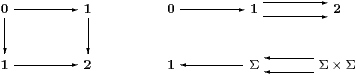The following results are based on the stronger assumption that this map is split epi, which it is in topology. It seems unlikely that any other application would require the weaker hypothesis.]

Lemma 11.3 The map 01 is a Σ-split mono iff Σ has a constant.

Proof    I01→Σ≡ Σ1.         ▫

If I≡ ⊤, this makes 0 a closed subspace; if I≡ ⊥ then it’s open (Examples 2.5). However, we shall call the constant ⋆ here to emphasise that we are not using any lattice structure. Then coproduct inclusions are also Σ-split monos:

Lemma 11.4     X≅{X+Y ∣ <π0,⋆>} and Y≅{X+Y ∣ <⋆,π1>}.

Proof    These idempotents arise from the diagram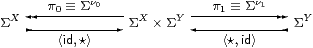▫

We want to show that every map U2 arises in this way from a unique coproduct. Because of the continuation-passing behaviour, it is convenient to treat 2 as a subspace of ΣΣ×Σ. Then the definable elements are π0≡λ x y.x and π1≡λ x y.y, which simply select the appropriate continuation from the pair.

Lemma 11.5     2 ≅  {ΣΣ×Σ ∣ λF F.F(Fπ0,Fπ1)}.

Proof    This is the primality equation, F PPx.F(λφ.φ x)), with λ x replaced by the two values 0,1:2, and φ:Σ2 by a pair.         ▫

We shall abuse our language by calling the two-argument functions PΣ×Σ that belong to this subspace “prime”. However, we need yet another characterisation of them.

Lemma 11.6     Γ⊢ PΣ×Σ is prime iff, for x,y,z:Σ and GΣΣ,

 P(x,x)   ⇔   x             P(P(x,y),z)   ⇔   P(x,z)   ⇔   P(x,P(y,z))
 G(λ z. P(z,⋆))   ⇔   P(Gid, G(λ z.⋆))      G(λ z. P(⋆,z))   ⇔   P(G(λ z.⋆), Gid).

Proof    If P is prime, so Γ, F2(Σ×Σ) ⊢ F P   ⇔   P(Fπ0,Fπ1), then

• put F≡λ Q.x, so F PFπ0Fπ1x and primality says that xP(x,x);
• put F≡λ Q.Q(Q(x,y),z), so Fπ0x, Fπ1z and P(P(x,y),z)≡F PP(x,z);
• put F≡λ F.Gz.F(z,⋆)), so Fπ0Gid, Fπ1Gz.⋆) and primality says that Gz.P(z,⋆))≡ F PP(GidGz.⋆)).

The converse is not needed in the following applications. We first put G≡λθ.Fx yP(x,y)), so Gz.⋆) ⇔ Fx y.⋆), GidF P,

 G(λ z.P(z,⋆))   ⇔   F(λ x y.P(P(x,y), ⋆))   ⇔   F(λ x y.P(x,⋆)),
 G(λ z.P(⋆,z))   ⇔   F(λ x y.P(⋆,P(x,y)))    ⇔   F(λ x y.P(⋆,y)),

with which the equations involving G give

 F(λ x y.P(x,⋆))   ⇔   P(F P, F(λ x y.⋆))      F(λ x y.P(⋆,y))   ⇔   P(F(λ x y.⋆),  F P).

Next put G≡ λθ.Fx yx), so

 G(λ z.⋆)   ⇔   F(λ x y.⋆),       G(λ z.P(z,⋆))    ⇔   F(λ x y.P(x,⋆))   and   Gid   ⇔   Fπ0,

with which the first G-equation gives

 F(λ x y.P(x,⋆))   ⇔    P(Fπ0,  F(λ x y.⋆)),

and similarly G≡ λθ.Fx yy) yields Fx y.P(⋆,y)) ⇔ P(Fx y.⋆), Fπ1).

From these four consequences of the higher-type G-equations, together with the ones of base type, we deduce that P is prime:

 F P ⇔ P(P(F P, F(λ x y.⋆)), P(F(λ x y.⋆),F P)) ⇔ P(F(λ x y.P(x,⋆)),     F(λ x y.P(⋆,y))) ⇔ P(P(Fπ0,  F(λ x y.⋆)),  P(F(λ x y.⋆),  Fπ1)) ⇔ P(Fπ0,Fπ1).         ▫

Proposition 11.7 Let u:UP uΣ×Σ be prime. Then the following subspaces are well defined, their coproduct is U and the squares are pullbacks.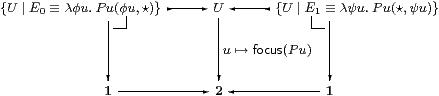Proof    To show that E0 is a nucleus, we must show for u:U and F3 U that

 E0(λ v.F(λφ.E0φ v)) u   ⇔   P u(F(λφ.P u(φ u,⋆)), ⋆)   ≡  P u(y,⋆)

is equal to

 E0(λ v.F(λφ.φ v)) u   ⇔   P u(F(λφ.φ u),  ⋆)   ≡  P u(x,⋆).

Put G≡λθ.F(λφ.θ(φ u)) in the Lemma. Then

 y ≡ F(λφ.P u(φ u,⋆)) ⇔ G(λ z.P u(z,⋆)) ⇔ P u(G id, G(λ z.⋆)) ⇔ P u(F(λφ.φ u),  F(λφ.⋆)) ≡ P u(x,t),

so P u(y,⋆) ⇔ P u(P u(x,t),⋆) ⇔ P u(x,⋆) as required.

To test that the left hand square is a pullback, we are given Γ⊢ v:U such that Γ⊢ P v0. Then

 Γ, θ:ΣU  ⊢     E0θ v   ⇔   P v(θ v,⋆)   ⇔   π0 (θ v,⋆)   ⇔   θ v,

as is required to form the mediator Γ⊢ admitv:{UE0}.

To show that U is the coproduct, we must show that ΣU is the associated product, i.e. that θ:ΣU corresponds bijectively to <φ,ψ> where φ=E0φ and ψ=E1ψ. In fact, P serves for the pairing operation as well as for the projections: θ≡λ u.P uuu).

 θ  ↦  <λ u.P u(θ u,⋆), λ u.P u(⋆,θ u)>  ↦  λ u.P u(P u(θ u,⋆), P u(⋆,θ u))  =  λ u.θ u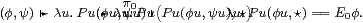▫

Theorem 11.8 If (S,Σ) is monadic and Σ has a constant then S is extensive, i.e. it has stable disjoint coproducts [Tay99, Section 5.5].Proof    We have to show that the commutative diagram is a pair of pullbacks iff its top row is a coproduct. The map U2 must arise from a prime u:UP uΣ×Σ, from which Proposition 11.7 constructs subspaces forming pullbacks and a coproduct. Since pullbacks are unique up to isomorphism, if the given diagram is a pair of pullbacks then it is isomorphic to that in the Proposition.

Conversely, if we are given a coproduct UX+Y then u:UP uΣ×Σ is defined by x:XP x≡π0 and y:YP y≡π1. Then E0 in the Proposition becomes

 E0θ  =  E0<φ,ψ>  =  <λ x.π0<φ,ψ> x,  λ y.⋆>  =  <φ,⋆>,

which agrees with Lemma 11.4.         ▫

Corollary 11.9 Let x:Xf x,g x:Z and x:XP xΣ×Σ with P prime. Then

 if  (focusP)  then  f x  else  g x   =   focus(λθ. P x (θ(f x)) (θ(g x))).         ▫

Turning from coproducts to coequalisers, of course we can only construct those that are Σ-split. We leave the reader to formulate Beck-style equations as in Section 3, concentrating instead on the analogue for quotients of the comprehension calculus for subspaces in Section 8. Beware also that the class of Σ-epis is stable under products but not necessarily pullbacks. For the topological motivation, recall that the quotient topology on a set Y induced by the function q:XY from a topological space has VY open iff q−1V is open in X.

Lemma 11.10 Let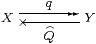be a Σ-split epi, and put RQ· q. Then

 Y  ≅  {Σ2 X ∣ E},   where EF F  ≡  F(λ x.F(λφ.R φ x)),
 q x  =  admit(λφ. Rφ x)   and   Q φ  =  λ y. i yφ.

Proof    Three of the four squarescommute by naturality, but that from Y to Σ2 X need not. So E is the composite from Σ2 X anticlockwise back to itself:

 E
≡  Σ2 q
 η
ΣY; ηYQ  =
 η
ΣXq; ηY; ΣQ  =
 η
ΣX; ηX; ΣΣq; ΣQ

so EF F  ⇔  ηΣXηX2 R F)) F  ⇔  Fx.F(λφ. Rφ x)). Next,

 i(q x)  =  ΣQ(ηY(q x))  =  ΣQ(Σ2 q(ηX x))  =  ΣR(ηX)  =  λφ. Rφ x,

whence q x = admit(λφ. Rφ x). Finally, i yφ  =  ΣQY y)φ  =  (λφ. φ y)(Qφ)  =  Qφ y.         ▫

Proposition 11.11 YX/R ,   q x ≡ [x] and

 (let  y=[x]  in  f x)  ≡  focus(λθ.Q(θ· f)y)  ≡  focus(λθ.(i y)(θ· f))

satisfy the rules

 x:X   ⊢   [x]:X/R           /I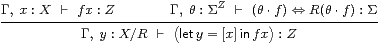/E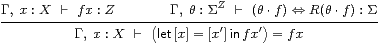/β
 y:X/R   ⊢   (let  y=[x]  in  [x]) = y          /η,

together with those for the quotient topology/ I, Σ/ E, Σ/ β and Σ/ η),

 φ:ΣX ⊢ Qφ:ΣX/R φ:ΣX,  x:X ⊢ (Qφ)(q x)   ⇔   Rφ x ψ:ΣX/R ⊢ Σqψ:ΣX ψ:ΣX/R ⊢ Q(Σqψ)  =  ψ.

Proof    We verify the /β and /η rules:

 let   [x]=[x′]  in  f x′ = focus(λθ.i(q x)(λ x′.θ(f x′)))     definition = focus(λθ.(λφ.Rφ x)(λ x′.θ(f x′)))     Lemma 11.10 = focus(λθ.R(θ· f) x) = focus(λθ.(θ· f) x)  =  f x      R(θ· f)=(θ· f) let  y=[x]  in  [x] = focus(λψ. (i y)(ψ· q)) = focus(λψ.Q(ψ· q)y)     Lemma 11.10 = focus(λψ.(Q·Σq)ψ y) = focus(λψ.ψ y)  =  y      Q·Σq=id.         ▫

Remark 11.12 Notice that the β-rule is not a computational reduction, but a denotational equation that is a consequence of the equation R(θ· f)=(θ· f) in its hypothesis. A compiler equipped with a proof assistant might perhaps be able know this, but otherwise we can only hope that the implementation will somehow find its way from one side of the β-rule to the other.

Let us specialise this to sets and (discrete) topological spaces, now making use of the lattice structure.

Example 11.13 Section C 10 constructs the quotient X/δ of an overt discrete object X by the open equivalence relation classified by δ:X× X→Σ. Overt discrete means that X is equipped with predicates ∃XX→Σ and (=X):X× X→Σ satisfying the usual properties.

The construction in Lemma C 10.8 obtains ΣX (which is unfortunately called ΣQ=E there, for “quotient” and “equaliser”) as a retract of ΣX, namely as the image of the closure operation

 R  ≡  Σq·∃q  ≡  λφ x.∃ y.δ(x,y)∧φ(y).

The quotient space is therefore X/δ=X/R={Σ2 XE}, where

 E F F ≡ F(λ x.F(λφ.∃ y.δ(x,y)∧ φ y)) q(x) ≡ admit(λφ.∃ y.δ(x,y)∧ φ(y)).

If f:XZ respects the equivalence relation δ then the β-rule is

 focus(λθ.∃ y.δ(x,y)∧θ(f y))  =  f x.

In principle this computation involves a search of the equivalence class, which makes sense, given the connection between coequalisers and while programs [Tay99, Section 6.4].

Example 11.14 As explained in Remark C 10.12, there is another representation of X/δ that is more like the familiar one with equivalence classes. It arises from the factorisationof the transpose of the equivalence relation. This generalises

ℕ  ≅  {Σ ∣ E}  by   n
 ⎧ ⎨ ⎩ n ⎫ ⎬ ⎭
,   where   E  ≡  λ Fφ.∃ n.F(λ m.m=n)∧φ n,

cfSection A 10. The quotient is now given by {ΣXE}, where

 E ≡ λ Fφ.∃ x.F(λ y.δ(x,y))∧φ x,

If f:XZ respects the equivalence relation then the mediator X/δ→ Z is

 y  ↦   focus(λθ.∃ x′.i y x′∧θ(f x′)).

This selects an element x′ from the equivalence class yX/δ (classified by a predicate i y∈ΣX) and applies f to it, as we would expect. Substitution of yq x yields the same formula focus(λθ.∃ x′.δ(x,x′)∧θ(f x′)) as before.         ▫

Remark 11.15 Here is a summary of the comprehension types that we have used.

 X ≅ {Σ2 X ∣ λF F.F(λ x.F(λφ.φ x))}     Remark 4.11 {X ∣ E0} × {Y ∣ E1} = {X× Y ∣ E1X· E0Y}     Proposition 5.12 {X ∣ E0}+{Y ∣ E1} = {ΣΣX×ΣY ∣ E}     Example 11.2 where   EH H = H<λ x.H(λφψ.E0φ x), λ y.H(λφψ.E1φ y)> Σ{X ∣ E} = {ΣX ∣ ΣE}      Proposition 6.2 {{X ∣ E1} ∣ E2} = {X ∣ E2}     Proposition 6.10 pts(A,α) = {ΣA ∣ λ Fφ.φ(α F)}     Example 11.1 X/R = {Σ2 X ∣ λF F.F(λ x.F(λφ.R φ x))}     Proposition 11.11 U⋂(X∖ V) = {X ∣ λφ.U∧φ∨ V}     Examples 2.5 X/δ = {Σ2 X ∣ λF F. F(λ x.F(λφ.∃ y.δ(x,y)∧ φ y))} ≅ {ΣX ∣ λ Fφ.∃ x.F(λ y.δ(x,y))∧φ x}     Examples 11.13f.